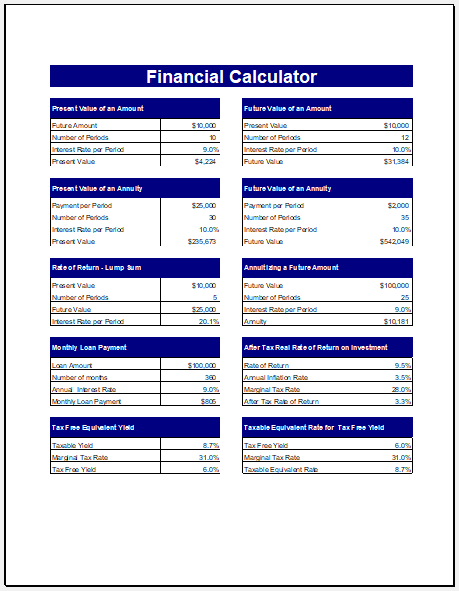# Financial Calculator Template

## What is a financial calculator?

The financial calculator is one of the essential accounting tools used to measure different parameters such as interest rate, annuity period, principal amount and a lot more. This calculator performs all the common functions that basically a business needs to carry out different operations successfully.

The financial calculator is a more advanced form of calculator which is more efficient than a standard calculator. Most of the financial calculators are user programmable that allow the user to customize the template.

## What are the benefits of using the financial calculator?

Different accounting industry professionals and students of accounting using the financial calculator are one of the primary tools. The reason why most of the accounting professionals and other people related to the field of accounting make use of a financial calculator is the ability of the calculator to calculate the net present value as well as an internal rate of return.

People who want to evaluate whether it is beneficial for them to invest in a particular project or not can use the financial calculator to quickly perform the task of evaluation. The financial calculator is also considered to be a useful tool because it enables the user to calculate the monthly annuity he is required to pay

## What is the basic purpose of using the financial calculator?

The basic purpose of using the financial calculator is to calculate the interest rate, present value, total payments to be made each month and a lot more. There are different types of financial calculators available. The user can choose the one depending on his personal preferences and needs. However, there are five functions which are common to every financial calculator. Another objective behind using the financial calculator is to determine the series of cash flow

## How to use the financial calculator?

In order to make effective use of the financial calculator, you should follow the following steps:

1. The first thing before you start using the financial calculator is to determine which variable you want to use the financial calculator since there are various variables that can be solved using it.
2. Enter the values required by the calculator to calculate the variable. Enter the amount and then the corresponding function key. For example, you can press ‘’N’’ button in order to mention the number of periods.
3. There is a ‘’solve’’ or ‘’compute’’ button on the calculator that solves the value of the given variable. All you need to do is press the button of the variable to be solved and then the compute button. The calculator will display the result on its screen

If you don’t want to use the financial calculator because you think it is more complex, you can simply make use of Microsoft Spreadsheet for this purpose. There are also many websites that provide the financial calculator template which is a readymade calculator. It requires the user to fill in the empty fields and it simply calculates everything on its own. You can either download the template or use it online.

PreviewFormat: MS Excel [.xlsx] 2007/2010
File Size: 87 KB